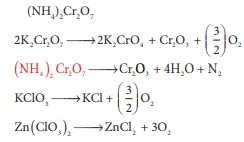# Unit IV: Transition and Inner Transition Elements - Online Test

Q1. Sc( Z=21) is a transition element but Zinc (z=30) is not because
Explaination / Solution:
No Explaination.

Q2. Which of the following d block element has half filled penultimate d sub shell as well as half filled valence sub shell?
Explaination / Solution:

Cr [Ar] 3d5 4s1

Q3. Among the transition metals of 3d series, the one that has highest negative ( M2+ /M) standard electrode potential is
Explaination / Solution:
No Explaination.

Q4. Which one of the following ions has the same number of unpaired electrons as present in V3+?
Explaination / Solution:
No Explaination.

Q5. The magnetic moment of Mn2+ ion is
Explaination / Solution:

Mn2+ => 3d5 contains 5 unpaired electrons

n=5 ; … = √n(n+2) BM

= √[5(5+2)] = √35 = BM

Q6. Which of the following compounds is colourless?
Explaination / Solution:

Ti4+ contains no unpaired electrons in d orbital, hence no d-d transition.

Q7. the catalytic behaviour of transition metals and their compounds is ascribed mainly due to
Explaination / Solution:
No Explaination.

Q8. The correct order of increasing oxidizing power in the series
Explaination / Solution:

VO2+ {+5} < Cr2O72− {+6} < MnO4 {+7}

greater the oxidation state, higher is the xidizing power

Q9. The alloy of copper that contain Zinc is
Explaination / Solution:

Brass contains 70% Cu + 30% Zn

Q10. Which of the following does not give oxygen on heating?
Explaination / Solution: